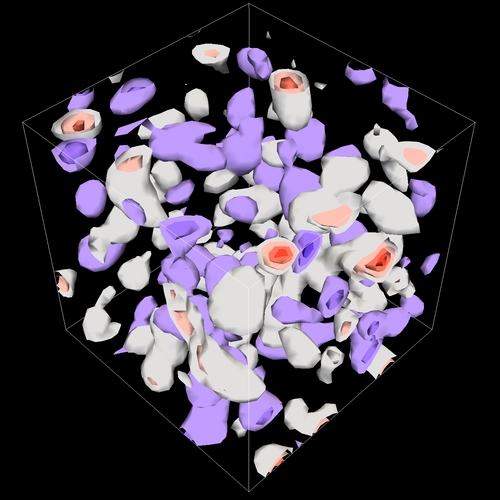Claudio Rebbi, Adam Avakian, Ron Babich, Richard Brower, Mike Clark, James Osborn, David Schaich

Calculations have been performed within the lattice formulation of Quantum Chromodynamics (QCD), exploring new physics while developing optimized code for these and future investigations. QCD describes the interactions of quarks and gluons, such as those that constitute the proton. In the lattice formulation, the quark and gluon fields are discretized and placed onto a four-dimensional grid. The resulting system, with millions of degrees of freedom, is simulated stochastically. These simulations require repeated inversion of the Dirac operator, conceptually a large sparse matrix, which is performed iteratively.

One focus of our current work is the comparison of QCD to superficially similar theories that may have significantly different observable behavior. These theories remain poorly understood due to the difficulty of the required calculations, and only in recent years has high-performance computing reached the point where such calculations can be performed.

We are also studying the contributions of “disconnected diagrams” to the strange quark content of the nucleon. This also represents a significant computational challenge, since disconnected diagrams require the evaluation of the trace of the inverted Dirac operator. An important component of our investigation is the development of novel multiscale methods that promise to reduce the variance in stochastic estimates of the trace.Contours of constant topological charge density for a quantum field theory on a lattice

#### Visualization Sequences

Contours of constant topological charge density for a quantum field theory on a lattice. (Courtesy C. Rebbi, A. Avakian, R. Babich, R. Brower, M. Clark, J. Osborn, D. Schaich, R. Gasser)

Isosurfaces of constant topological charge density for a quantum field theory on a lattice. (Courtesy C. Rebbi, A. Avakian, R. Babich, R. Brower, M. Clark, J. Osborn, D. Schaich, R. Gasser)

Volume rendering of constant topological charge density for a quantum field theory on a lattice. (Courtesy C. Rebbi, A. Avakian, R. Babich, R. Brower, M. Clark, J. Osborn, D. Schaich, R. Gasser)